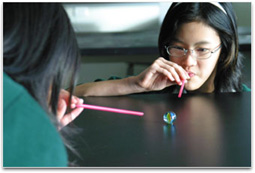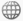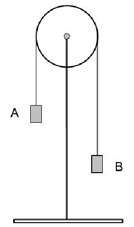# Newton's understanding of forces and motion

This focus idea is explored through:

## Contrasting student and scientific views

### Student everyday experiencesIt is very difficult to tell with the naked eye if an object is accelerating (for example, a ball flying through the air) and so students do not tend to think of motion in terms of whether it is accelerated or not. Accelerated motion is also a difficult concept for students because it occurs when an object changes either speed or direction or both. Students are familiar with objects accelerating from rest or braking, for example a car speeding up or to a lesser extent slowing down at the traffic lights. However, it is much more difficult to determine if an object already moving is changing its speed unless the change is dramatic. Students seldom identify a car turning a corner at constant speed as accelerating because their common understanding requires the object to be changing speed for it to be accelerating.

Even senior students regularly confuse ‘acceleration’ and ‘speed’; for example they may think that if speed is increasing then acceleration is also increasing.

Research: Champagne, Klopfer & Anderson (1980), Trowbridge & McDermott (1981), Loughran, Berry & Mulhall (2006)

Students often have a set of ‘intuitive rules’ that seem to explain everyday examples of motion (see the teaching ideas on forces and motion at the lower levels). These rules appear to work when students ignore friction and air resistance; friction and air resistance are generally not seen by students as involving forces.

These ideas are also discussed in the focus idea Friction is a Force.

Research: Mitchell (2007)

A common view that persists at this level is that a moving object must have a force acting on them in the direction of their motion. Some students also strongly believe that this force is being used up if the object is slowing down. This may be partly a terminology problem: what students label as a force in these situations is similar to what scientists call ‘momentum’.

Research: Champagne, Klopfer & Anderson (1980), Gunstone & Watts (1985), Gunstone, Mulhall & McKittrick (2007), Osborne & Freyberg (1985)

Students often struggle to grasp the concept of net force, and often think it is an extra force in addition to the actual forces on an object.

Research: Gunstone, Mulhall & McKittrick (2007)

### Scientific view

The net force is the combined effect (the sum) of the real forces acting on the object. Net force is a valuable construct that has no separate existence of its own, unlike the real forces acting on the object, i.e. it is not an additional force.

Research: Gunstone, Mulhall & McKittrick (2007)

While the net force on an object is zero, its speed and direction of motion remain unchanged (and stationary objects remain stationary). See Newton’s first law of motion.

When there is a net force on an object, it causes the object to accelerate in the direction of the net force; this is not the same as the direction of the motion unless the object is going in a straight line. The magnitude of the net force on the object is the product of its mass and its acceleration (Newton’s second law of motion).

## Critical teaching ideas

• An object accelerates when its speed changes or its direction of motion changes or both.
• Changes in an object’s speed are always continuous even though in some situations it may appear to be almost instantaneous (for example a golf ball when hit by a golf club, or car collisions).
• The net force on an object is the combined effect (the sum) of all the pushing and pulling forces actually acting on the object.
• If the forces pushing or pulling on an object are not balanced (a net force acts) then the object will accelerate in the direction of the net force.

Research: Loughran, Berry & Mulhall (2006), Gunstone, Mulhall & McKittrick (2007)Explore the relationships between ideas about force and acceleration in the Concept Development Maps – Laws of Motion

The ideas about forces and motion below contribute to student understanding of this topic. These are each covered in greater detail in the sequence of teaching ideas introduced at the lower levels:

• A net force on an object changes its motion – the greater the net force, the greater the acceleration. More massive objects require bigger net forces to accelerate the same amount as less massive objects.
• A force is described by using the expression ‘force of A on B’ and drawing an arrow to show the direction of the force. For example, the weight of a book is the ‘force of Earth on the book’. It can be represented diagrammatically by an arrow drawn through the centre of the book directed downwards towards the Earth.

It is important for students to have a sound qualitative understanding of the ideas of Newton’s second law before mathematics is introduced. Students need exposure to situations that require verbal explanations about the forces involved before they are exposed to quantitative work involving formulae.

A simple mathematical relationship exists between the mass of an object (m), the net force on the object (f) and its acceleration (a). The acceleration of an object is directly proportional to the net force and indirectly proportional to the object’s mass (a = f/m).

## Teaching activities

### Opening up discussion via a shared experience

Students should use the expression ‘force of A on B’ to identify the agent and receiver of a force in various situations, and use an arrow to represent the force’s direction. They can then be asked to identify all the actual forces and the net force on objects in a wide range of motion contexts. Some examples include: a ball rolling along a table; a ball that was rolling on a table but is now stopped; a ball thrown up in the air that is moving upwards (or downwards); a skateboard rider moving down a gently sloping path at a constant speed.

In particular, the role of friction needs to be explored because it has such a huge influence on the observed motion of everyday objects.

Ideas about friction are explored in the focus idea Friction is a force.

### Open up discussion via a shared experiencePOE (Predict-Observe-Explain) can be used to develop powerful understandings about balanced and unbalanced forces. Using a bicycle wheel mounted as a pulley, with a bucket of sand hanging from either side, students can respond to each question below. They should observe what happens and then explain their observation:

• With one bucket (A) higher than the other (B), and both held stationary, ask the students which weighs more.
• With A pulled down so it is level with B, ask students to predict what will happen when you let go of A.
• The same as set up as above, but with a much heavier weight (so it will cause movement). Ask students whether B’s speed is the same at two widely separated points in its path.
• Explore the effects of adding and subtracting large and small weights while the buckets are moving. There are several possibilities here which can foster greater student engagement if they are encouraged to suggest some of these new possibilities.

Research: Loughran, Berry & Mul​hall (2006)

### Challenge existing ideas

P.O.E Place a puck on air table and ask students to predict how they would keep it moving at a steady speed by pushing on it with a ruler. Most students are surprised that it is impossible to achieve a steady speed with this action and are intrigued as any amount of push with the ruler makes the puck keep 'running away from them'.

### Promote reflection on and clarification of existing ideas

Road safety is a topic where students can explore the issues associated with mass and speed of vehicles involved in car accidents and how these may influence the injuries inflicted on passengers.

Encourage students to think of the advantages of introducing light weight vehicles and the disadvantages of being involved in collisions with much heavier trucks.

The Digilearn objects below provide some simulations students can experiment with.

### Promote reflection on how student ideas have changed

Students can look for examples in films and cartoons where Newton’s laws are not obeyed. The popular ‘Coyote and the Road Runner’ cartoons use frequent scenes where students’ alternative conceptions are displayed. Identify situations and discuss these in class.

### Collect evidence/data for analysis

Data loggers can be used to record and graph students’ movement and the motion of other objects. Utilise software programs that can analyse video (or digitally recorded) motion.

## Further resources

Science related interactive learning objects can be found on the FUSE Teacher Resources page.

To access the interactive learning object below, teachers must login to FUSE and search by Learning Resource ID:

• Accelerate - Pilot a spaceship between planets. Use Newton's second law of motion to work out the acceleration needed in a series of challenges. Drive at a constant acceleration, then make adjustments for cargo and friction. Examine velocity-time line graphs showing acceleration as the gradient. This learning object is a combination of three learning objects in the same series.
Learning Resource ID: UM9P74
• It’s a drag – Students investigate the braking efficiency of cars and trucks. They test stopping distances under controlled conditions and compare effects of vehicle type, speed, tyres, road surface and weather conditions.
Learning Resource ID:  W2XXLR